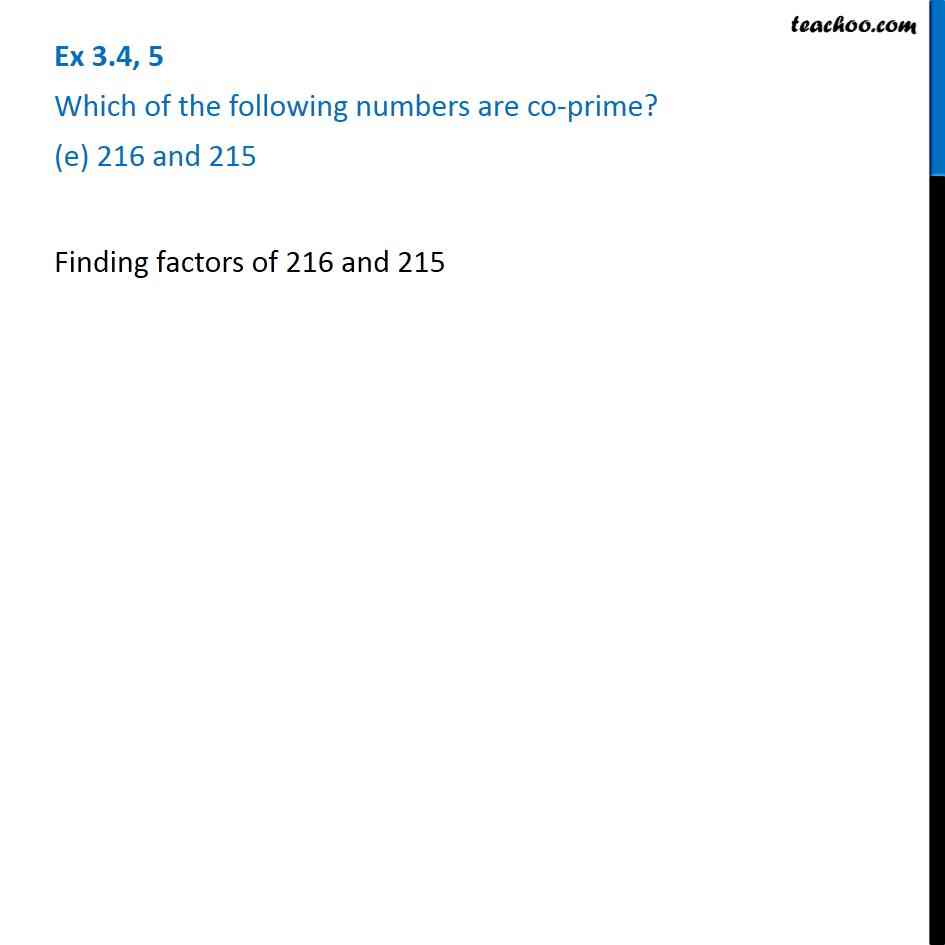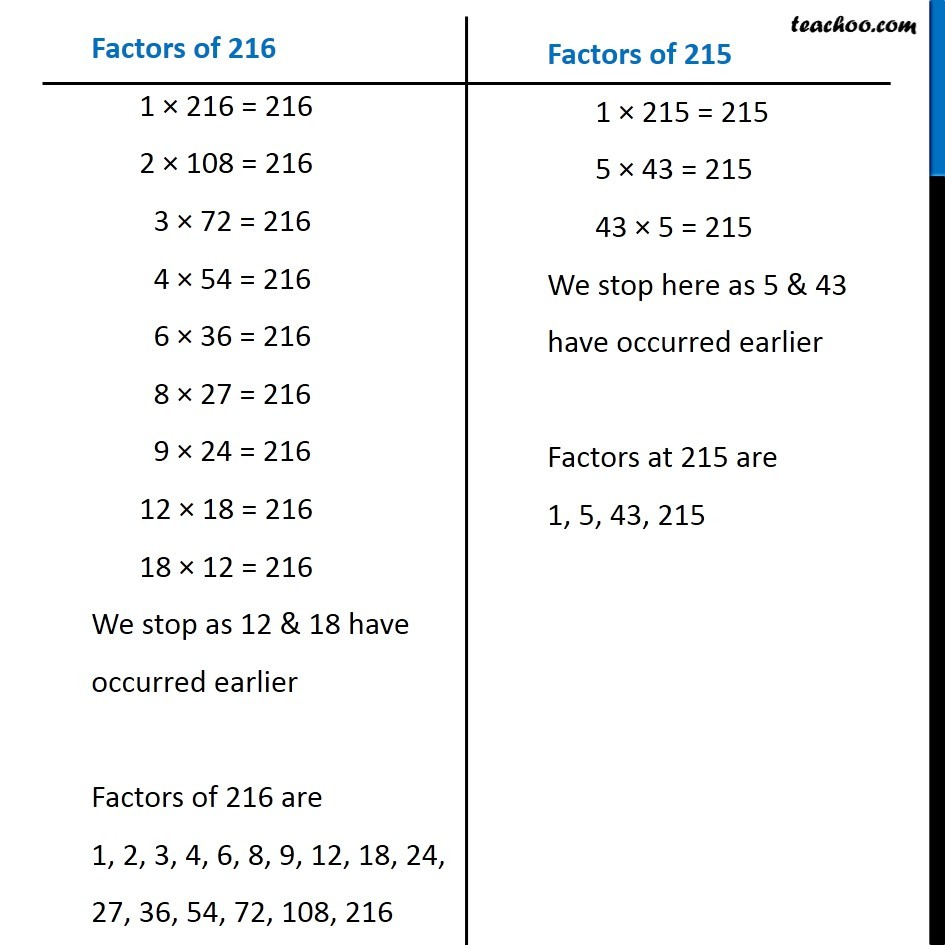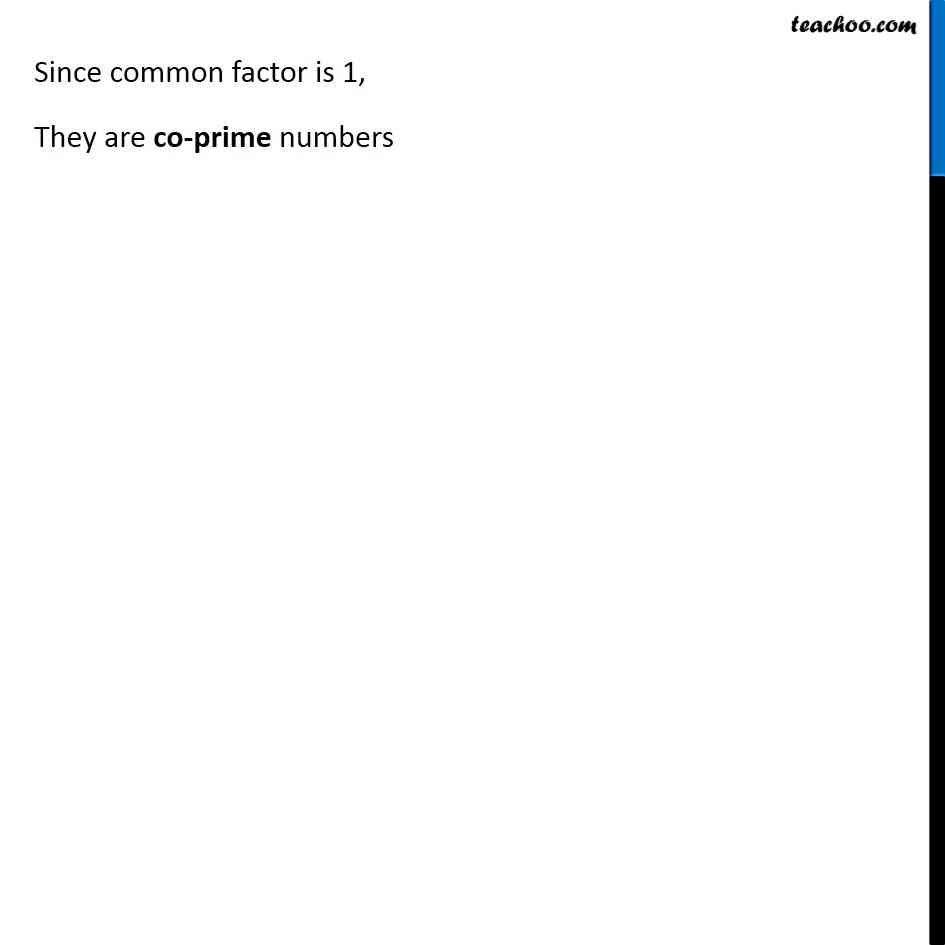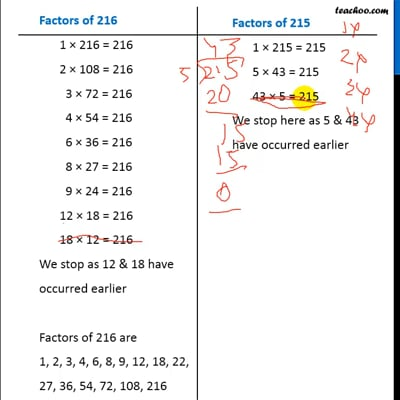Ex 3.4

Chapter 3 Class 6 Playing with Numbers
Serial order wiseThis video is only available for Teachoo black users

Introducing your new favourite teacher - Teachoo Black, at only ₹83 per month

### Transcript

Ex 3.4, 5 Which of the following numbers are co-prime? (e) 216 and 215 Finding factors of 216 and 215 Factors of 216 1 × 216 = 216 2 × 108 = 216 3 × 72 = 216 4 × 54 = 216 6 × 36 = 216 8 × 27 = 216 9 × 24 = 216 12 × 18 = 216 18 × 12 = 216 We stop as 12 & 18 have occurred earlier Factors of 216 are 1, 2, 3, 4, 6, 8, 9, 12, 18, 22, 27, 36, 54, 72, 108, 216 Factors of 215 1 × 215 = 215 5 × 43 = 215 43 × 5 = 215 We stop here as 5 & 43 have occurred earlier Factors at 215 are 1, 5, 43, 215 Since common factor is 1, They are co-prime numbers